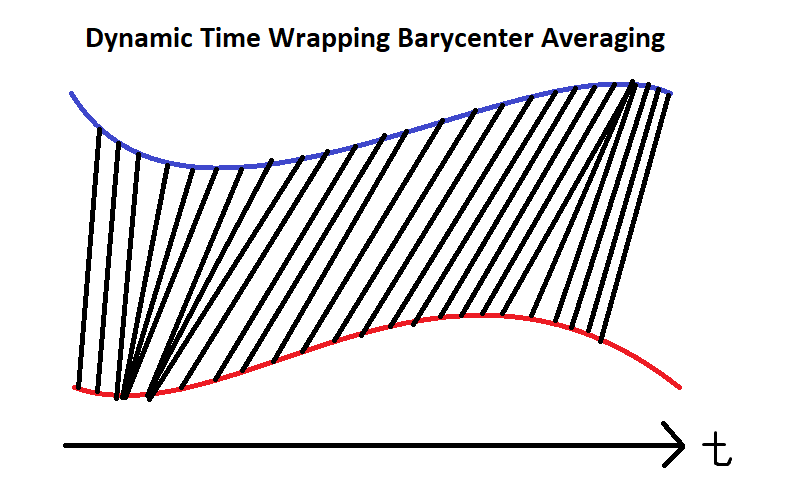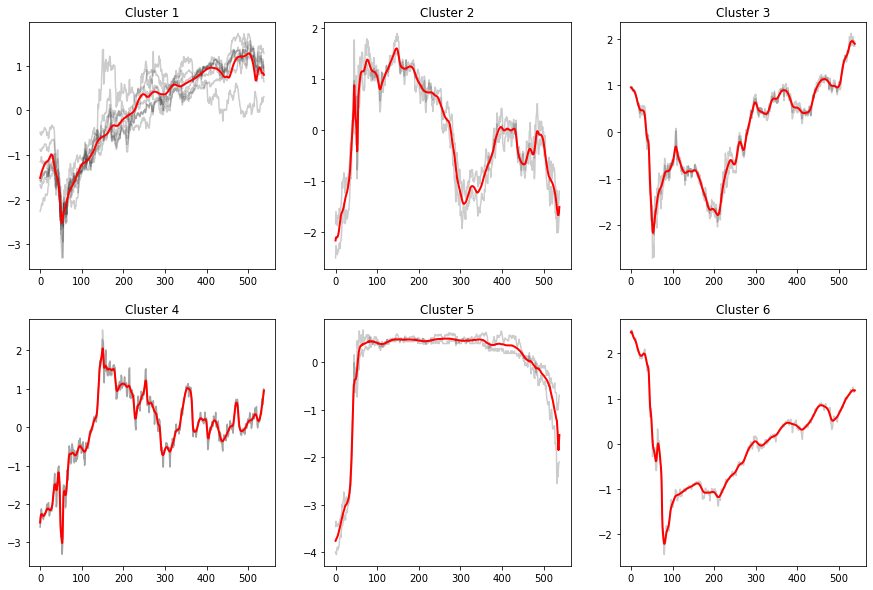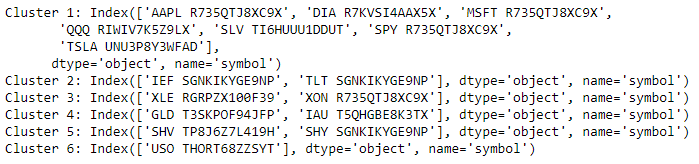# Machine Learning

## Tslearn

### Introduction

This page explains how to build, train, test, and store tslearn models.

### Import Libraries

Import the tslearn libraries.

from tslearn.barycenters import softdtw_barycenter
from tslearn.clustering import TimeSeriesKMeans

### Get Historical Data

Get some historical market data to train and test the model. For example, get data for the securities shown in the following table:

Group NameTickers
Overall US marketSPY, QQQ, DIA
Tech companiesAAPL, MSFT, TSLA
Long-term US Treasury ETFsIEF, TLT
Short-term US Treasury ETFsSHV, SHY
Heavy metal ETFsGLD, IAU, SLV
Energy sectorUSO, XLE, XOM
qb = QuantBook()
tickers = ["SPY", "QQQ", "DIA",
"AAPL", "MSFT", "TSLA",
"IEF", "TLT", "SHV", "SHY",
"GLD", "IAU", "SLV",
"USO", "XLE", "XOM"]
symbols = [qb.AddEquity(ticker, Resolution.Daily).Symbol for ticker in tickers]
history = qb.History(symbols, datetime(2020, 1, 1), datetime(2022, 2, 20))

### Prepare Data

You need some historical data to prepare the data for the model. If you have historical data, manipulate it to train and test the model. In this example, standardize the log close price time-series of the securities. Follow these steps to prepare the data:

1. Unstack the historical DataFrame and select the close column.
2. close = history.unstack(0).close
3. Take the logarithm of the historical time series.
4. log_close = np.log(close)

Taking the logarithm eases the compounding effect.

5. Standardize the data.
6. standard_close = (log_close - log_close.mean()) / log_close.std()

### Train Models

Instead of using real-time comparison, we could apply a technique call Dynamic Time Wrapping (DTW) with Barycenter Averaging (DBA). Intuitively, it is a technique of averaging a few time-series into a single one without losing much of their information. Since not all time-series would move efficiently like in ideal EMH assumption, this would allow similarity analysis of different time-series with sticky lags. Check the technical details from tslearn documentation page.We then can separate different clusters by KMean after DBA.

# Set up the Time Series KMean model with soft DBA.
km = TimeSeriesKMeans(n_clusters=6,   # We have 6 main groups
metric="softdtw",  # soft for differentiable
random_state=0)

# Fit the model.
km.fit(standard_close.T)

### Test Models

We visualize the clusters and their corresponding underlying series.

1. Predict with the label of the data.
2. labels = km.predict(standard_close.T)
3. Create a class to aid plotting.
4. def plot_helper(ts):
# plot all points of the data set
for i in range(ts.shape):
plt.plot(ts[i, :], "k-", alpha=.2)

# plot the given barycenter of them
barycenter = softdtw_barycenter(ts, gamma=1.)
plt.plot(barycenter, "r-", linewidth=2)
5. Plot the results.
6. j = 1
plt.figure(figsize=(15, 10))
for i in set(labels):
# Select the series in the i-th cluster.
X = standard_close.iloc[:, [n for n, k in enumerate(labels) if k == i]].values

# Plot the series and barycenter-averaged series.
plt.subplot(len(set(labels)) // 3 + (1 if len(set(labels))%3 != 0 else 0), 3, j)
plt.title(f"Cluster {i+1}")
plot_helper(X.T)

j += 1

plt.show()7. Display the groupings.
8. for i in set(labels):
print(f"Cluster {i+1}: {standard_close.columns[[n for n, k in enumerate(labels) if k == i]]}")### Store Models

You can save and load tslearn models using the Object Store.

#### Save Models

Follow these steps to save models in the Object Store:

1. Set the key name of the model to be stored in the Object Store.
2. model_key = "model"
3. Call the GetFilePath method with the key.
4. file_name = qb.ObjectStore.GetFilePath(model_key)

This method returns the file path where the model will be stored.

5. Call the to_hdf5 method with the file path.
6. km.to_hdf5(file_name + ".hdf5")

You must save a model into the Object Store before you can load it from the Object Store. If you saved a model, follow these steps to load it:

1. Call the ContainsKey method.
2. qb.ObjectStore.ContainsKey(model_key)

This method returns a boolean that represents if the model_key is in the Object Store. If the Object Store does not contain the model_key, save the model using the model_key before you proceed.

3. Call the GetFilePath method with the key.
4. file_name = qb.ObjectStore.GetFilePath(model_key)

This method returns the path where the model is stored.

5. Call the from_hdf5 method with the file path.
6. loaded_model = TimeSeriesKMeans.from_hdf5(file_name + ".hdf5")

This method returns the saved model.

### Reference

• F. Petitjean, A. Ketterlin, P. Gancarski. (2010). A global averaging method for dynamic time warping, with applications to clustering. Pattern Recognition. 44(2011). 678-693. Retreived from https://lig-membres.imag.fr/bisson/cours/M2INFO-AIW-ML/papers/PetitJean11.pdf
• You can also see our Videos. You can also get in touch with us via Discord.